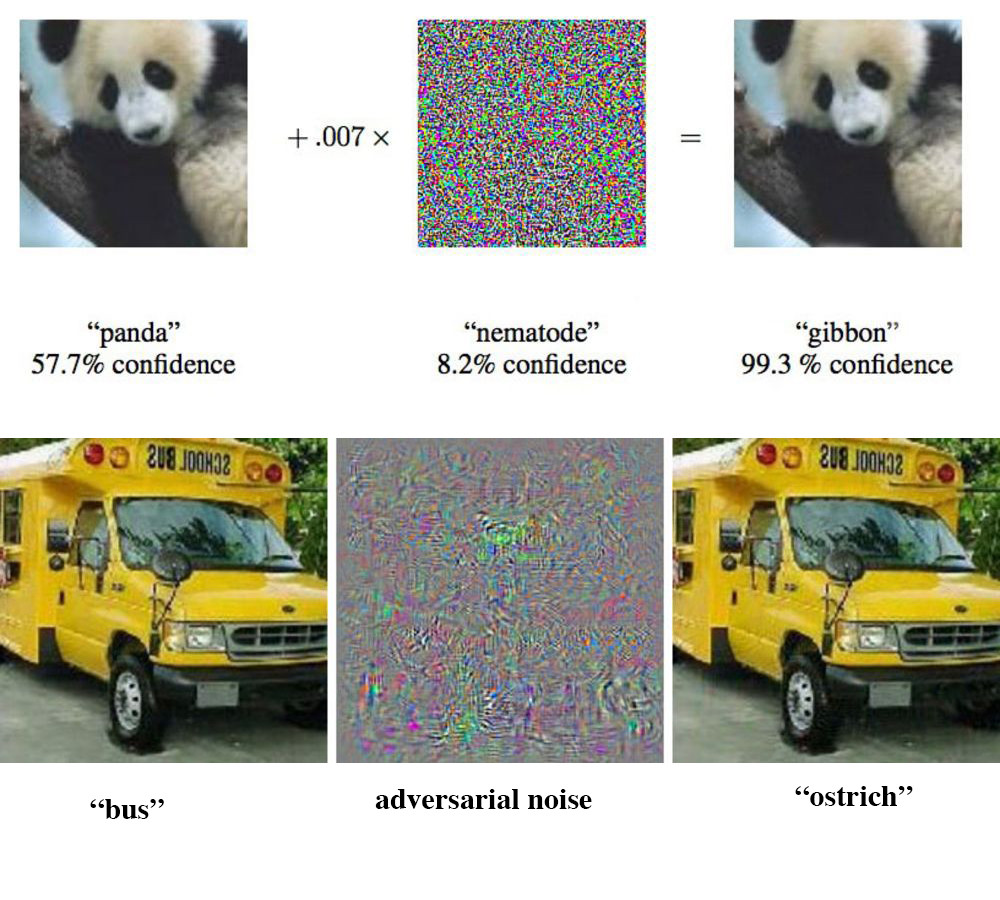# Statistical Robustness for Automated Driving Software

Van C. Ngo · March 16, 2021

There are many uncertainties in automated driving systems. For example:

• unpredictable environments such as highways and urban areas
• limitations and noise of sensor data such as cameras and Lidars
• unpredictable actuation such as the drive-by-wire hardware
• inaccurate motion model
• stochastic features of algorithms such as localization, perception and timing properties

Thus, robustness w.r.t these uncertainties is very important factor. It provides measures that are used for safely deploying the system and giving public trust.

The following are some examples of robustness:

• During 1 hour of operation, is the probability that the object detection produces different output on a perturbed input smaller than $$10^{-9}$$?
• During 1 hour of operation, is the probability that the Monte-Carlo localization produces significant difference vehicle pose on noised Lidar data smaller than $$10^{-9}$$?
• During 1 hour of operation, is the probability that the latency of sending any message is within 10 ms smaller than $$10^{-9}$$?Note that $$10^{-9}$$ is the required failure rate of the ISO-26262 ASIL D. To compute those probabilities, we need to have at least one billion hours of operation or around thirty billion miles of driving. Obviously, it is absolutely impossible in practices even in simulation mode.

In our new research project, we provide a framework for automatically estimating these very small probabilities (rare events).

• It reasons about how robust a software module is w.r.t uncertainty, called statistical robustness
• The estimation run-time is in the order of several hours
• The software module can be highly trained ML models, localization, or any software’s timing properties

The impact is providing a quantitative measures about the software robustness in order to

• make safety analysis possible and
• make automated driving software accepted more easily by public authorities